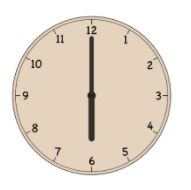Courses
Courses for Kids
Free study material
Offline Centres
MoreLast updated date: 05th Dec 2023
Total views: 291.5k
Views today: 5.91k

# Find the angle between minute hand and hour hand at 6 O’clock.Verified
291.5k+ views
Hint: A clock has a shape of the circle made up of ${{360}^{{}^\circ }}$, as a clock has 12 hours, each hour will draw an angle measure of ${{30}^{{}^\circ }}$.

Our task is to find the angle between minute hand and hour hand at 6 O’clock.
These types of problems are called clock angle problems where we must find the angle between the hands of an analog clock at a specific time mentioned.
Generally, an analog clock that shows us time is divided into 12 sections, as there are 12 numbers on the clock that represents the hours.Keeping this in mind we can say that each number represents a definite angle.
As it is divided into 12 equal parts, the measure of the angle between each number is given as:
$\dfrac{360}{12}=30$.
Thus, we can further say that, each hour will make an angle measure of ${{30}^{{}^\circ }}$in a clock.
Similarly, since there are 60 minutes in any clock, let us find out the angle measure by each minute.
The angle measure per minute is given as:
$\dfrac{360}{60}=6$
Therefore, it makes an angle measure of ${{6}^{{}^\circ }}$ for every minute in the clock.
Now, we were supposed to find the angle made by the minute and hour hand at 6 O’clock.
So, when the time is 6 O’clock, we have six hours in between, where each hour makes an angle measure of ${{30}^{{}^\circ }}$.
Therefore, we have:
$\left( 6 \right)\left( 30 \right)=180$
Therefore,${{180}^{{}^\circ }}$ is the angle made by the hour and minute hand of the clock at 6 O’clock.

Note: Alternatively, we can illustrate a diagram representing the time of 6 O’clock and observe that the minute hand and hour hand make a straight line, so we can directly say that the angle is ${{180}^{{}^\circ }}$, since it is a straight line. ${{180}^{{}^\circ }}$angle is also called a straight angle.